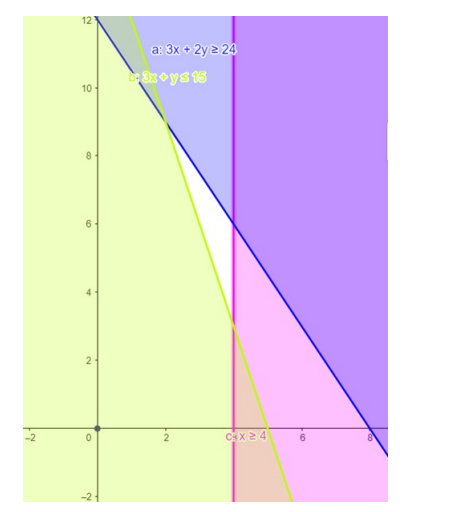# Solve the given inequalities

Question:

Solve the given inequalities $3 x+2 y \geq 24,3 x+y \leq 15, x \geq 4$, graphically in two - dimensional plane.

Solution:

The graphical representation of $3 x+2 y \geq 24,3 x+y \leq 15$

$x \geq 4$ is given by common region in the figure below.

$3 x+2 y \geq 24 \ldots \ldots$ (1)

$3 x+y \leq 15 \ldots \ldots$ (2)

$x \geq 4 \ldots \ldots$ (3)

Inequality (1) represents the region above line $3 x+2 y=24$ (including the line $3 x+2 y=24$ ).

Inequality $(2)$ represents the region below line $3 x+y=15$ (including the line $3 x+y=15$ ).

Inequality (3) represents the region in front of line $x=4$ (including the line $x=4$ ).

Therefore, we can see in the figure that there is no common shaded region.

So there linear inequalities in equations has no solution.

This can be represented as follows,